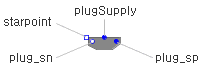Annulus Pipe - MapleSim Help

Annulus Pipe

Lossy model of an annulus pipeDescription The Annulus Pipe component models an annulus pipe with losses. The pressure drop is computed with the Darcy equation. When the Reynolds number is greater than the maximum value for laminar flow but less than the minimum value for turbulent flow, the friction factor is determined using linear interpolation.Equations $q={q}_{A}=-{q}_{B}=\mathrm{Re}A\frac{\mathrm{\nu }}{{\mathrm{D}}_{h}}$ $p={p}_{A}-{p}_{B}=\frac{1}{2}L\mathrm{\rho }\frac{{\mathrm{\nu }}^{2}}{{\mathrm{D}}_{h}^{3}}\mathrm{Re}\left\{\begin{array}{cc}{f}_{\mathrm{Colebrook}}\left(\left|\mathrm{Re}\right|,\frac{\mathrm{\epsilon }}{{\mathrm{D}}_{h}}\right)\left|\mathrm{Re}\right|\phantom{\rule[-0.0ex]{6.0ex}{0.0ex}}& \mathrm{mode}=\mathrm{+turbulent}\vee \mathrm{mode}=\mathrm{-turbulent}\\ \left({f}_{L}+\frac{{f}_{T}-{f}_{L}}{{\mathrm{Re}}_{T}-{\mathrm{Re}}_{L}}\left(\left|\mathrm{Re}\right|-{\mathrm{Re}}_{L}\right)\right)\left|\mathrm{Re}\right|& \mathrm{mode}=\mathrm{+mixed}\vee \mathrm{mode}=\mathrm{-mixed}\\ \mathrm{AnnulusShapeFactor}\left({\mathrm{D}}_{i},{\mathrm{D}}_{0}\right)\phantom{\rule[-0.0ex]{12.5ex}{0.0ex}}& \mathrm{otherwise}\end{array}$ $\mathrm{mode}=\left\{\begin{array}{cc}\mathrm{+turbulent}& {\mathrm{Re}}_{T}<\mathrm{Re}\\ \mathrm{-turbulent}& {\mathrm{Re}}_{T}<-\mathrm{Re}\\ \mathrm{+mixed}\phantom{\rule[-0.0ex]{2.0ex}{0.0ex}}& {\mathrm{Re}}_{L}<\mathrm{Re}\\ \mathrm{-mixed}\phantom{\rule[-0.0ex]{2.0ex}{0.0ex}}& {\mathrm{Re}}_{L}<-\mathrm{Re}\\ \mathrm{laminar}\phantom{\rule[-0.0ex]{3.0ex}{0.0ex}}& \mathrm{otherwise}\end{array}$ ${\mathrm{D}}_{h}={\mathrm{D}}_{o}-{\mathrm{D}}_{i}\phantom{\rule[-0.0ex]{6.0ex}{0.0ex}}A=\frac{\mathrm{\pi }}{4}\left({\mathrm{D}}_{o}^{2}-{\mathrm{D}}_{i}^{2}\right)$ ${f}_{\mathrm{Colebrook}}=\left(\mathrm{Re},{\mathrm{\epsilon }}_{\mathrm{D}}\right)\to {\left(1.8{{\mathrm{log}}_{10}\left(\frac{6.9}{\mathrm{Re}}+\left(\frac{{\mathrm{\epsilon }}_{\mathrm{D}}}{3.7}\right)\right)}^{1.11}\right)}^{-2}$Variables

 Name Units Description Modelica ID $p$ $\mathrm{Pa}$ Pressure drop from A to B p ${p}_{X}$ $\mathrm{Pa}$ Pressure at port X pX $q$ $\frac{{m}^{3}}{s}$ Flow rate from A to B q ${q}_{X}$ $\frac{{m}^{3}}{s}$ Flow into port X qXConnections

 Name Description Modelica ID $\mathrm{portA}$ Upstream hydraulic port portA $\mathrm{portB}$ Downstream hydraulic port portBParametersGeneral Parameters

 Name Default Units Description Modelica ID ${\mathrm{D}}_{i}$ $0.005$ $m$ Annulus pipe inner diameter Di ${\mathrm{D}}_{o}$ $0.01$ $m$ Annulus pipe outer diameter Do $\mathrm{\epsilon }$ $1.5·{10}^{-5}$ $m$ Height of inner surface roughness epsilon $L$ $5$ $m$ Length of pipe L ${\mathrm{Re}}_{L}$ $2·{10}^{3}$ Reynolds number at transition to laminar flow ReL ${\mathrm{Re}}_{T}$ $4·{10}^{3}$ Reynolds number at transition to turbulent flow ReT